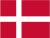### Dish Cloth 24

Cast on 60 stitches and knit 4 rows and then pattern until the cloth has the desired length. Then knit 4 rows and bind off.

1.row: 5 p, 1 k, 1 p, 1 k, 1 p, 1 k
2.row: 1 k, 1 p, 1 k, 1 p, 6 k
3.row: as row 1
4.row: as row 2
5.row: as row 1
6.row: as row 2
7.row: as row 1
8.row: as row 2
9.row: 1 p, 1 k, 1 p, 1 k, 1 p, 5 k
10.row: 6 p, 1 k, 1 p, 1 k, 1 p
11.row: as row 9
12.row: as row 10
13.row: as row 9
14.row: as row 10
15.row: as row 9
16.row: as row 10

 X X 16 X X X 15 X X 14 X X X 13 X X 12 X X X 11 X X 10 X X X 9 X X X X X X X X 8 X X X X X X X 7 X X X X X X X X 6 X X X X X X X 5 X X X X X X X X 4 X X X X X X X 3 X X X X X X X X 2 X X X X X X X 1
You have to remember that all uneven rows start in the right side of the diagram and X=purl and ▢= knit. All equal rows start in the left side of the diagram and X=knit and ▢=purl.Danish